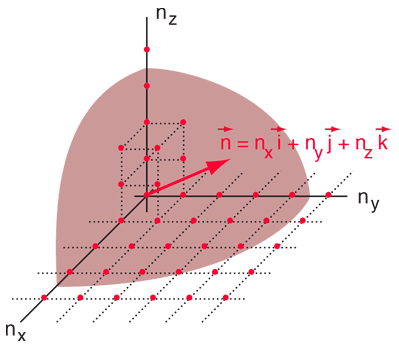# A calculation of microstates

• I
• Kashmir
Kashmir
*Pathria, Statistical mechanics pg 11,4ed*

The text is long but it is straightforward. The question is at last about the equation given at end

In order to find the number of microstates ##\Omega(N,V,E##) author writes

" In other words, we have to determine the total number of (independent) ways of satisfying the equation
##
\sum_{r=1}^{3 N} \varepsilon_{r}=E,
"##
Where ##E## is the total energy of system and
##\varepsilon_{r}## is the energy of ##r##th degree of freedom.

" Now, the energy eigenvalues for a free, nonrelativistic particle confined to a cubical box of side ##L\left(V=L^{3}\right)##, under the condition that the wave function ##\psi(\boldsymbol{r})## vanishes everywhere on the boundary, are given by
##
\varepsilon\left(n_{x}, n_{y}, n_{z}\right)=\frac{h^{2}}{8 m L^{2}}\left(n_{x}^{2}+n_{y}^{2}+n_{z}^{2}\right) ; \quad n_{x}, n_{y}, n_{z}=1,2,3, \ldots,
##
where ##h## is Planck's constant and ##m## the mass of the particle. The number of distinct eigenfunctions (or microstates) for a particle of energy ##\varepsilon## would, therefore, be equal to the number of independent, positive-integral solutions of the equation
##
\left(n_{x}^{2}+n_{y}^{2}+n_{z}^{2}\right)=\frac{8 m V^{2 / 3} \varepsilon}{h^{2}}=\varepsilon^{*} .
##
We may denote this number by ##\Omega(1, \varepsilon, V)##. Extending the argument, it follows that the desired number ##\Omega(N, E, V)## would be equal to the number of independent, positiveintegral solutions of the equation
##
\sum_{r=1}^{3 N} n_{r}^{2}=\frac{8 m V^{2 / 3} E}{h^{2}}=E^{*}
##"

"... the number ##\Omega(N, V, E)##, or better ##\Omega_{N}\left(E^{*}\right)## is equal to the number of positiveintegral lattice points lying on the surface of a ##3 N##-dimensional sphere of radius ##\sqrt{E}^{*} ## . The number ##\Sigma_{N}\left(E^{*}\right)##, which denotes the number of positive-integral lattice points lying on or within the surface of a ##3 N##-dimensional sphere of radius ##\sqrt{E}^{*}##. In terms of our physical problem, this would correspond to the number, ##\Sigma(N, V, E)##, of microstates of the given system consistent with all macrostates characterized by the specified values of the parameters ##N## and ##V## but having energy less than or equal to ##E##

##\Sigma(N, V, E)=\sum_{E^{\prime} \leq E} \Omega\left(N, V, E^{\prime}\right)
##
or
##
\Sigma_{N}\left(E^{*}\right)=\sum_{E^{*} \leq E^{*}} \Omega_{N}\left(E^{{*\prime}}\right)##

"...let us examine the behavior of the numbers ##\Omega_{1}\left(\varepsilon^{*}\right)## and ##\Sigma_{1}\left(\varepsilon^{*}\right)## which correspond to the case of a single particle confined to the given volume ##V##. The number ##\Sigma_{1}\left(\varepsilon^{*}\right)## on the other hand, exhibits a much smoother asymptotic behavior. **From the geometry of the problem, we note that, asymptotically, ##\Sigma_{1}\left(\varepsilon^{*}\right)## should be equal to the volume of an octant of a three-dimensional sphere of radius** ##\sqrt{\varepsilon}^{*}##, **that is**,
##
\lim _{\varepsilon^{*} \rightarrow \infty} \frac{\Sigma_{1\left(\varepsilon^{*}\right)}}{(\pi / 6) \varepsilon^{* 3 / 2}}=1.
##

* Why is the above equation true?

Homework Helper
Hi,

If I understand your question, you agree with the bold-faced statement and ask about the equation ?

Well, the volume of a sphere is ##{4\over 3} \pi r^3##, so an octant has a volume 1/8 of that.

##\ ##

Kashmir
Hi,

If I understand your question, you agree with the bold-faced statement and ask about the equation ?

Well, the volume of a sphere is ##{4\over 3} \pi r^3##, so an octant has a volume 1/8 of that.

##\ ##
hi, no I don't understand the statement.

Mentor
You have to imagine what it looks like in n-space: consider each state as a point ##(n_x, n_y, n_z)## in three-dimensional space, with corresponding energy ##\varepsilon(n_x, n_y, n_z)##.

Asymptotically, these states become so close to one another than one can consider ##n_x##, ##n_y##, and ##n_z## as continuous instead of discrete. In that case, all states with the same energy are characterized by a vector ##\vec{n} = (n_x, n_y, n_z)## of the same length. That vector can point anywhere in the octant where ##n_x##, ##n_y##, and ##n_z## are all positive. The tip of that vector describes (1/8th of) a spherical shell, and the total number of states is the volume of (1/8th of) the corresponding sphere.(Picture "borrowed" form http://hyperphysics.phy-astr.gsu.edu/hbase/quantum/phodens.html)

•vanhees71, Kashmir and BvU
Kashmir
You have to imagine what it looks like in n-space: consider each state as a point ##(n_x, n_y, n_z)## in three-dimensional space, with corresponding energy ##\varepsilon(n_x, n_y, n_z)##.

Asymptotically, these states become so close to one another than one can consider ##n_x##, ##n_y##, and ##n_z## as continuous instead of discrete. In that case, all states with the same energy are characterized by a vector ##\vec{n} = (n_x, n_y, n_z)## of the same length. That vector can point anywhere in the octant where ##n_x##, ##n_y##, and ##n_z## are all positive. The tip of that vector describes (1/8th of) a spherical shell, and the total number of states is the volume of (1/8th of) the corresponding sphere.
View attachment 299200
(Picture "borrowed" form http://hyperphysics.phy-astr.gsu.edu/hbase/quantum/phodens.html)
This cleared it a lot. Thank you.
I have a doubts regarding what you said:

1) Why do "...Asymptotically these states become so close to one another...? How do we know that they become so close?

Mentor
1) Why do "...Asymptotically these states become so close to one another...? How do we know that they become so close?
I was using the same wording as in your book, but this will depend on exactly how you are taking the thermodynamic limit. For instance, taking ##V \rightarrow \infty##, you have ##L \rightarrow \infty## in the equation for energy and the energy levels become continuous. Also, for ##\vec{n}## big enough, the discreteness of ##n_x##, ##n_y##, and ##n_z## becomes negligible.

Kashmir
The tip of that vector describes (1/8th of) a spherical shell, and the total number of states is the volume of (1/8th of) the corresp
Why should the total number of states be equal to the volume of an octant?

Once we assume that the number of states is continuous it follows that they are infinite in number.

Last edited: